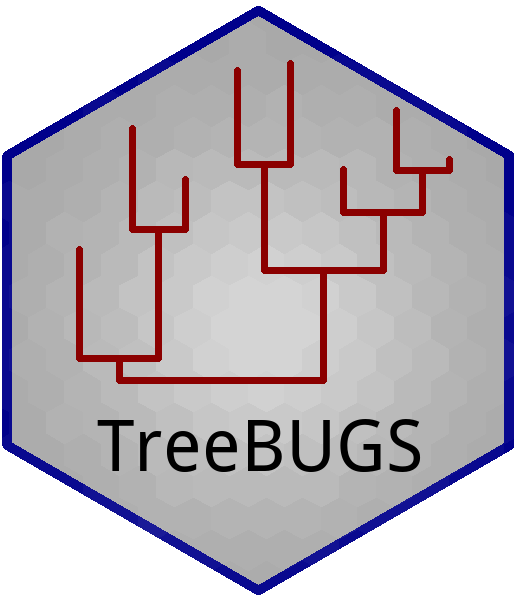# TreeBUGS: Introduction to Hierarchical MPT Modeling

## General Procedure of Using TreeBUGSIn the most simple scenario, the following steps are required:

1. Define path to existing MPT model file in .eqn format (cf. multiTree; Moshagen, 2010)
2. Define path to data set with individual frequencies (.csv file: comma separated, rows=persons, columns=labeled categories)
3. Call one of the fitting functions betaMPT or traitMPT (examples below)
4. Check convergence of MCMC chains
5. Summarize and plot results

In the following, these steps are explained in more detail. Note that TreeBUGS requires a recent version of the software JAGS (http://mcmc-jags.sourceforge.net/).

### 1. Step: MPT model file in EQN syntax

The model needs to be passed in the standard .eqn file format (e.g., as in multiTree; Moshagen, 2010). As an example, consider the most simple two-high-threshold model (2HTM), each line defines a single processing path containing tree label, category label, and model equations:

#####Title: 2HTM
Target  Hit    Do
Target    Hit    (1-Do)*g
Target    Miss   (1-Do)*(1-g)
Lure      FA     (1-Dn)*g
Lure      CR     (1-Dn)*(1-g)
Lure      CR     Dn

Note that category labels (e.g., hit, miss,…) must start with a letter (different to multiTree or HMMTree) and match the column names of . The model equations require the multiplication sign * and parameters should not be summarized, e.g., by a^2*(1-a). As an input for TreeBUGS, the model file (e.g., "2htm.txt") needs to be saved in the current working directory. Otherwise, the relative or absolute path to the file must be specified (e.g., models/2htm.txt). To check how TreeBUGS interprets a given .eqn-file, use:

readEQN(file = "pathToFile.eqn",       # relative or absolute path
restrictions = list("Dn=Do"),  # equality constraints
paramOrder = TRUE)             # show parameter order

Equality restrictions on the MPT parameters can either be provided in a list:

restrictions = list("Dn=Do", "g=0.5")

or by a path to a text file on the hard drive (e.g., restrictions="pathToFile.txt") that contains the equality constraints, one per row:

Dn=Do
g=0.5

### 2. Step: Data Set with Individual Frequencies

Data can be loaded from a comma-separated text file (.csv) in the following format:

Hit,   Miss,     FA,    CR
20,      10,      5,    25
13,       7,      9,    21
15,       5,      6,    14
.....


Note that the first line contains the category labels, which must match the category labels from the .eqn-file. The remaining rows contain individual frequencies. Similarly as for the .eqn file, the path to the data file can either be specified as data_ind.csv if it is in the current working directory, or as an relative or absolute path (e.g., "C:/models/data_ind.csv").

When using TreeBUGS within R, a data.frame or matrix with appropriate column names that match the category labels can be provided.

### 3. Step: Fit Hierarchical MPT Model

An hierarchical Beta-MPT model is fitted with the following code:

# load the package:
library(TreeBUGS)

# fit the model:
fitHierarchicalMPT <- betaMPT(
eqnfile="2htm.txt",         # .eqn file
data="data_ind.csv",        # individual data
restrictions=list("Dn=Do"), # parameter restrictions (or path to file)

### optional MCMC input:
n.iter = 20000,             # number of iterations
n.burnin = 5000,            # number of burnin samples that are removed
n.thin = 5,                 # thinning rate of removing samples
n.chains = 3                # number of MCMC chains (run in parallel)
)

A latent-trait model is fitted similarly by replacing betaMPT by traitMPT.

### 4. Step: Check convergence of MCMC chains

The functions betaMPT and traitMPT return a list that includes the original samples from the MCMC sampler for convergence checks. The MCMC samples are stored in fittedModel$runjags$mcmc as an mcmc.list object (see the package for an overview of convergence diagniostics). TreeBUGS provides a handy wrapper to access the most important plotting functions:

# Default: Traceplot and density
plot(fitHierarchicalMPT,    # fitted model
parameter ="mean"      # which parameter to plot
)
# further arguments are passed to ?plot.mcmc.list

# Auto-correlation plots:
plot(fitHierarchicalMPT,  parameter = "mean", type = "acf")

# Gelman-Rubin plots:
plot(fitHierarchicalMPT,  parameter = "mean", type = "gelman")

See and for further convergence statistics and plots. Note that inferences from the model can be invalid if the Markov-Chain Monte-Carlo (MCMC) sampler did not converge!

### 5. Step: Summarize and Plot Results

TreeBUGS produces an MPT-tailored summary of parameter estimates and convergence statistics:

• Information about the parameter posterior distribution: Mean, SD, Median, 2.5% and 97.5% quantiles
• Convergence: $$\hat R$$-Statistic (should be close to 1.00 for all parameters, e.g., $$\hat R < 1.05$$) and number of effective samples when accounting for auto-correlation (should be large)

To obtain the summary after fitting the model, simply use:

summary(fitHierarchicalMPT)

The following functions allow to plot parameter estimates, distributions, goodness of fit, and raw frequencies:

plotParam(fitHierarchicalMPT,     # estimated parameters
includeIndividual=TRUE  # whether to plot individual estimates
)
plotDistribution(fitHierarchicalMPT)      # estimated hierarchical parameter distribution
plotFit(fitHierarchicalMPT)               # observed vs. predicted mean frequencies
plotFit(fitHierarchicalMPT, stat = "cov") # observed vs. predicted covariance
plotFreq(fitHierarchicalMPT)              # individual and mean raw frequencies per tree
plotPriorPost(fitHierarchicalMPT)         # comparison of prior/posterior (group level parameters)

Parameter estimates (posterior mean, median, SD) can be extracted and saved to a file by using:

# matrix for further use within R:
tt <- getParam(fitHierarchicalMPT, parameter="theta",
stat="mean")
tt

# save complete summary of individual estimates to file:
getParam(fitHierarchicalMPT, parameter = "theta",
stat = "summary", file = "parameter.csv")